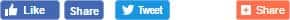• Mobile Apps

எஸ்.எஸ்.சி., எம்.டி.எஸ்., தேர்வு மாதிரி வினா - விடைஎழுத்தின் அளவு:

பதிவு செய்த நாள்

21 பிப்
2017
00:00

Quantitative Aptitude
55. What should be added to 16 to get -31? a) 15 b) -15 c) 47 d) -47
56. If the ratio of boys to girls in a class is B and the ratio of girls to boys is G, then 3(B + G) is? a) Equal to 3 b) Less than 3 c) More than 3 d) Loss than 1/3
57. A bag contains one rupee, 50 paise and 25 paise coins in the ratio 2 : 3 : 5, their total value is Rs. 114 the value of 50 paise coins is a) 24/- b) 36/- c) 48/- d) 72/-
58. There pots have the same volume. The ratio of milk and water in first, second and third pots are 3:2, 7:3 and 11:4 respectively. If the liquid of 3 pots are mixed, then the ratio of milk and water in the mixture is. a) 61:29 b) 61:30 c) 5:4 d) 29:61
59. What percent of a day in there hours? a) 12 ½ % b) 25% c) 80% d) 100/3 %
60. Two numbers are in the ratio 5:9 after adding 9 to each number; the ratio becomes 16:27. One number out of the two will be. a) 99 b) 77 c) 88 d) 66
61. Rs.700/- are distributed among A, B and C such that A gets half of what B gets and B gets half of what C gets. What is the share of C? a) Rs.600/- b) Rs.400/- c) Rs.300/- d) Rs.200/-
62. Kranti started a business investing Rs.9000/-,after 5 months Sudhakar joined with a capital of Rs.8000/-. If at the end of the year, they earn a profit of Rs.9870/- then what will be the share of Sudhakar in the profit? a) Rs.3690/- b) Rs.1883.78/- c) Rs.2380/- d) Rs.3,864/-
63. A number gets reduced to its one-third when 48 is subtracted from it. What is two-third of that number a) 24 b) 72 c) 36 d) 48
64. A number lies between the cubes of 15 and 16. If the number is divisible by the square of 12 as well as by 7 what is the number. a) 3469 b) 4032 c) 4045 d) 5249
65. If “m” and “n” are integers and mn =10 which of the following cannot be a value of (m + n) a) 25 b) 52 c) 101 d) 50
66. In an examination, 35% of the students passed and 455 failed. The total number of students who appeared for the examination was: a) 845 b) 1300 c) 490 d) 700
67. 248 + 15 + 169 is equal to a)16 b) 15 c)18.8 d) 16.6
68. A batsman makes a score of 87 runs in the 17th inning and thus increases his average by 3. Find his average after 17th inning. a) 30 b) 35 c) 38 d) 39
69. Three numbers are in the ratio of 4:5:6 and their average is 25. the largest number is a) 30 b) 32 c) 36 d) 42
70. The average age of five numbers of a family is 21 years. If the age of the grandfather was included, the average is increased by 9 years. The age of the grand father is.a) 66 years b) 72 years c) 75 years d) 84 years.

Answers55) d 56) c 57) b 58) a 59) a 60) a 61) c 62) c 63) d 64) b 65) d 66) d 67) a 68) d 69) a 70) b
தொடரும்

வாசகர்களுக்கு ஓர் அன்பான வேண்டுகோள்.
1.செய்திகள் குறித்த கருத்துக்களைப் பதிவு செய்யும்போது, எவருடைய மனதையும் புண்படுத்தாத வகையில், நாகரிகமான முறையில் உங்கள் கருத்துகள் இருக்க வேண்டும் என்று எதிர்பார்க்கிறோம்.
வாசகர் கருத்து
முதல் நபராக கருத்து தெரிவியுங்கள்!
உங்கள் கருத்தைப் பதிவு செய்ய :